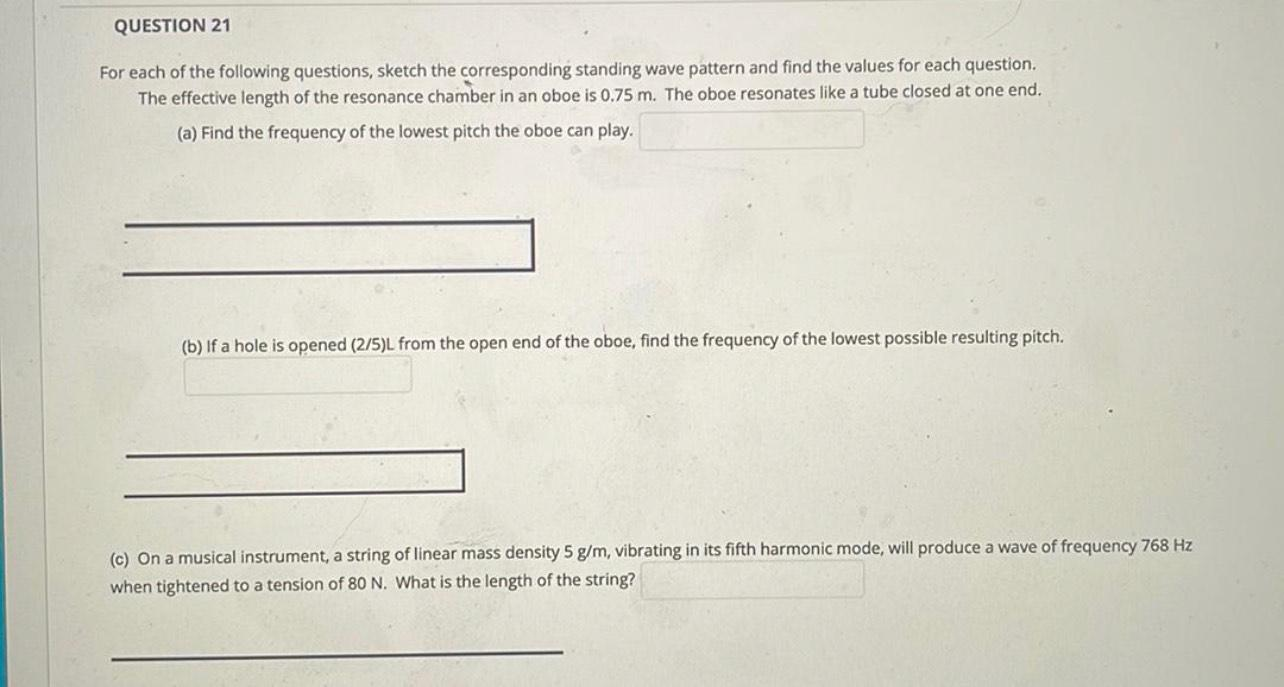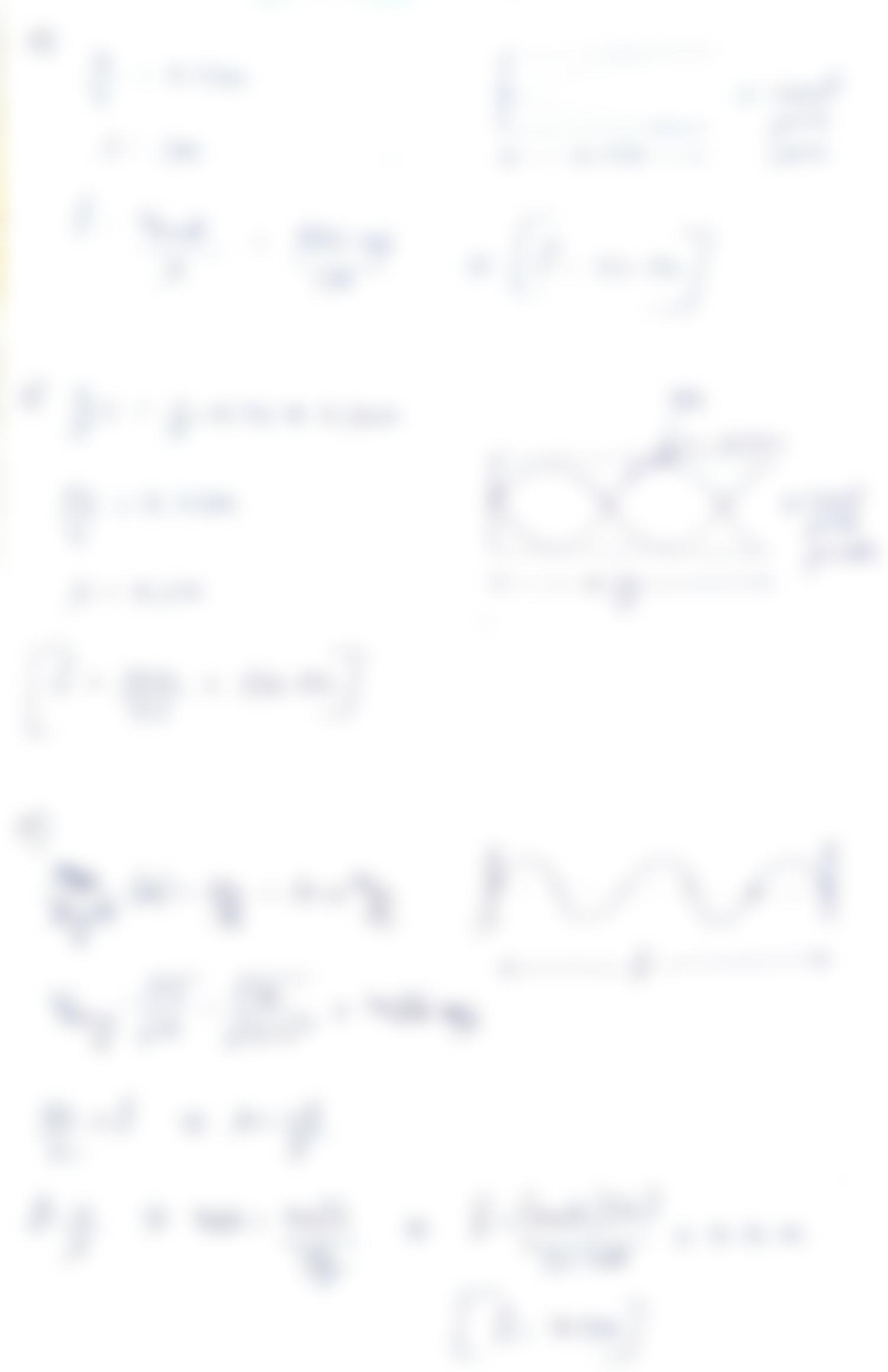Question:

QUESTION 21 For each of the following questions, sketch the corresponding standing wave pattern and find the values for each queQUESTION 21 For each of the following questions, sketch the corresponding standing wave pattern and find the values for each question. The effective length of the resonance chamber in an oboe is 0.75 m. The oboe resonates like a tube closed at one end. (a) Find the frequency of the lowest pitch the oboe can play. (b) If a hole is opened (2/5)L from the open end of the oboe, find the frequency of the lowest possible resulting pitch. (c) On a musical instrument, a string of linear mass density 5 g/m, vibrating in its fifth harmonic mode, will produce a wave of frequency 768 Hz when tightened to a tension of 80 N. What is the length of the string?